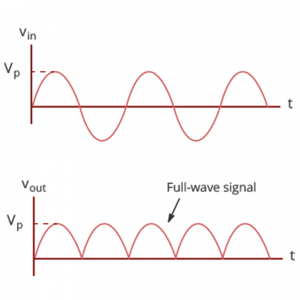# In-Depth Guide to Full Wave Rectifier

Electric circuits that convert alternating current to direct current are known as rectifiers. There are two types of rectifiers they are half-wave rectifiers and full-wave rectifiers. A half-wave rectifier loses a significant amount of power and is thus unsuitable for applications that require a smooth and continuous supply. We employ full-wave rectifiers for a smoother and more consistent supply.

## What is a Full Wave Rectifier?

A Full Wave Rectifier is a rectifier that transforms both halves of each alternating wave cycle (Alternating Current) into a pulsing DC (Direct Current) signal.

Full-wave rectifiers are used for a smoother and more consistent supply of power. Full-wave rectifiers are used to convert a whole cycle of alternating current voltage (AC) to direct current voltage (DC).

The full wave rectifier circuit can be built in two ways. The first technique involves a transformer with a central tap and two diodes. This design is known as a center-tapped full-wave rectifier.

The second approach employs a conventional transformer with four diodes placed in a bridge configuration. This is referred to as a bridge rectifier. In the next part, we will solely cover the center-tapped full wave rectifier. You may learn about bridge rectifiers in depth by reading our page on bridge rectifiers.

## Full Wave Rectifier Circuit Diagram## Full Wave Rectifier Waveform## Full Wave Rectifier Working

The full wave rectifier’s input AC is exceptionally high. The rectifier circuit’s step-down transformer transforms high-voltage alternating current to low-voltage alternating current. The anode of the center-tapped diodes is connected to the secondary winding of the transformer and the load resistor. The top half of the secondary winding becomes positive during the positive half cycle of the alternating current, while the second half of the secondary winding turns negative.

Diode D1 is forward biassed during the positive half cycle because it is linked to the top of the secondary winding. In contrast, diode D2 is reverse biassed since it is connected to the bottom of the secondary winding. As a result, diode D1 will conduct as a short circuit, and D2 will not conduct as an open circuit.

Since the top half of the secondary circuit becomes negative, and the bottom half of the circuit becomes positive during the negative half cycle, diode D1 is reverse biassed and diode D2 is forward biassed. Hence, DC voltage is achieved in full wave rectifiers for both positive and negative half cycles.

## Full Wave Rectifier Formulae

These are some characteristics of the Full-Wave rectifier

#### Peak Inverse Voltage

Peak inverse voltage is the greatest voltage that a diode can endure when reverse-biased before breaking down. The full-wave rectifier’s peak inverse voltage is twice that of a half-wave rectifier.

Where, $$D_{1} + D_{2}=2 V_{max}$$

#### DC Output Voltage

$$V_{dc} = V_{dc} R_{L} = V_{avg} R_{L}$$

#### RMS Value of Current

The current RMS value may be determined using the following formula:

$$I_{RMS} = \frac{ I_{max} }{ \sqrt{2} }$$

#### Form Factor

The full wave rectifier’s form factor is computed using the following formula:

$$K_{f} = \frac{RMS value of current}{Average value of Current}= \frac{ I_{rms} }{ I_{dc} }= \frac{ \frac{ I_{max} }{ \sqrt{2} } }{ \frac{2 I_{max} }{ \pi } }= \frac{ \pi }{2 \sqrt{2} } =1.11$$

#### Peak Factor

The peak factor of a full wave rectifier is given by the following formula:

$$K_{p} = \frac{Peak value of current}{RMS value of Current}= \frac{ I_{max} }{ \frac{ I_{max} }{ \sqrt{2} } }= \sqrt{2}$$

#### Rectification Efficiency

The following formula may be used to calculate the rectification efficiency of a full-wave rectifier:

$$\eta = \frac{DC output power}{AC Output Power}$$

Where the efficiency of the full wave rectifier is 81.2%.

## Pros and Cons of Full Wave Rectifier

#### Advantages of Full Wave Rectifier

• The ripple frequency of a full-wave rectifier is double the input frequency.
• The high output DC power.
• The ripple factor is lower.
• Increased output voltage.
• A higher factor of utilization for transformers.
• Easier to offer smooth ripple frequency.

• More intricate than a half-wave rectifier.
• More diodes are required: two for the center tap rectifier and four for the bridge rectifier.
• The diode has a higher PIV rating.
• Higher PIV diodes are greater in size and significantly more expensive.
• The center tap transformer is expensive.
• The center tap on the secondary winding of this rectifier is difficult to detect.
• When just a modest voltage needs to be rectified, the full-wave rectifier circuit is ineffective.

## Final Notes

Tutoroot’s online interactive classes will help you learn more about Full Wave Rectifier. We have skilled teachers, who are IIT alumni to assist you in your learning journey. Transform the way you learn with tutoroot.

## FAQ’s

What is Full wave Rectifier Efficiency?

A full-wave rectifier has a rectifier efficiency of 81.2%.

Ripple factor of a full wave rectifier?

The ripple factor of a full wave rectifier is 0.482.

What is a Full wave rectifier peak inverse voltage?

A Full Wave rectifier’s peak inverse voltage is 2Vm.